Test: Stress & Strain - 1

# Test: Stress & Strain - 1 - Mechanical Engineering

Test Description

## 20 Questions MCQ Test GATE Mechanical (ME) 2024 Mock Test Series - Test: Stress & Strain - 1

Test: Stress & Strain - 1 for Mechanical Engineering 2023 is part of GATE Mechanical (ME) 2024 Mock Test Series preparation. The Test: Stress & Strain - 1 questions and answers have been prepared according to the Mechanical Engineering exam syllabus.The Test: Stress & Strain - 1 MCQs are made for Mechanical Engineering 2023 Exam. Find important definitions, questions, notes, meanings, examples, exercises, MCQs and online tests for Test: Stress & Strain - 1 below.
Solutions of Test: Stress & Strain - 1 questions in English are available as part of our GATE Mechanical (ME) 2024 Mock Test Series for Mechanical Engineering & Test: Stress & Strain - 1 solutions in Hindi for GATE Mechanical (ME) 2024 Mock Test Series course. Download more important topics, notes, lectures and mock test series for Mechanical Engineering Exam by signing up for free. Attempt Test: Stress & Strain - 1 | 20 questions in 60 minutes | Mock test for Mechanical Engineering preparation | Free important questions MCQ to study GATE Mechanical (ME) 2024 Mock Test Series for Mechanical Engineering Exam | Download free PDF with solutions
 1 Crore+ students have signed up on EduRev. Have you?
Test: Stress & Strain - 1 - Question 1

### If a body is stressed within its elastic limit, the lateral strain bears a constant ratio to the linear strain. This constant is known as:

Detailed Solution for Test: Stress & Strain - 1 - Question 1

Poisson's ratio is the ratio of transverse strain to longitudinal strain.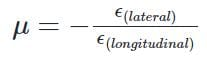For perfectly isotropic elastic material, Poisson’s ratio is 0.25 but for most of the materials, the value of Poisson's ratio lies in the range of 0 to 0.5.

Poisson's ratio for various materials are:

• Cork: 0.0
• Aluminium: 0.31
• Cast iron: 0.21 – 0.26
• Steel: 0.27 – 0.30
• Stainless steel: 0.30 – 0.31
• Copper: 0.33
• Rubber: 0.5
Test: Stress & Strain - 1 - Question 2

### Which point on the stress strain curve occurs after proportionality limit?

Detailed Solution for Test: Stress & Strain - 1 - Question 2

A stress-strain curve represents the relationship between the stress applied to a material and the resulting strain (deformation) experienced by the material. The curve can be divided into several regions, each corresponding to a different behavior of the material under stress.

1. Proportionality limit: This is the region where the stress-strain curve is linear, and the material follows Hooke's Law, which states that the stress is proportional to the strain. In this region, the material will return to its original shape and size when the stress is removed.

2. Elastic limit: This is the point on the stress-strain curve immediately after the proportionality limit. Up to the elastic limit, the material will still return to its original shape and size when the stress is removed, but the relationship between stress and strain is no longer linear. Beyond the elastic limit, the material will enter the plastic region, where it will experience permanent deformation even when the stress is removed.

3. Lower yield point: This is the point on the stress-strain curve where the material starts to yield or undergo plastic deformation. The material will not return to its original shape and size when the stress is removed at this point.

4. Upper yield point: This is the point on the stress-strain curve where the material has reached its maximum resistance to plastic deformation. Beyond this point, the material will continue to deform with little or no increase in stress.

5. Ultimate point: This is the point on the stress-strain curve where the material experiences its maximum stress before failure. Beyond this point, the material will begin to fracture and eventually break under the applied stress.

Since the elastic limit occurs immediately after the proportionality limit, it is the correct answer.

Test: Stress & Strain - 1 - Question 3

### A rod of length L and diameter D is subjected to a tensile load P. Which of the following is sufficient to calculate the resulting change in diameter?

Detailed Solution for Test: Stress & Strain - 1 - Question 3
• For longitudinal strain we need Young's modulus and for calculating transverse strain we need Poisson's ratio.
• We may calculate Poisson's ratio from E = 2G(1+μ) for that we need Shear modulus.
Test: Stress & Strain - 1 - Question 4

A static load is mounted at the centre of a shaft rotating at uniform angular velocity. This shaft will be designed for

Detailed Solution for Test: Stress & Strain - 1 - Question 4

When shaft rotates at constant ω, each fibre of the shaft will undergo tensile and compressive loads. Therefore, the shaft is under the action of cyclic load.Hence the design should be for fatigue loading.

Test: Stress & Strain - 1 - Question 5

Which of the following is a good example of a composite bar?

Detailed Solution for Test: Stress & Strain - 1 - Question 5

Composite Bars

• A composite bar is made of two bars of different materials rigidly fixed together.
• The strain in both bars /materials is the same under external load.
• Since strains in the two bars are the same, the stresses in the two bars/materials depends on their young’s modulus of elasticity.
• Examples are Reinforced cement concrete, Flitched beam.

RCC is a composite material made of steel and concrete in which concrete's relatively low tensile strength is compensated for by the inclusion of steel bars having higher tensile strength as reinforcement.

Test: Stress & Strain - 1 - Question 6

A 1.5 mm thick sheet is subject to unequal biaxial stretching and the true strains in the directions of stretching are 0.05 and 0.09. The final thickness of the sheet in mm is

Detailed Solution for Test: Stress & Strain - 1 - Question 6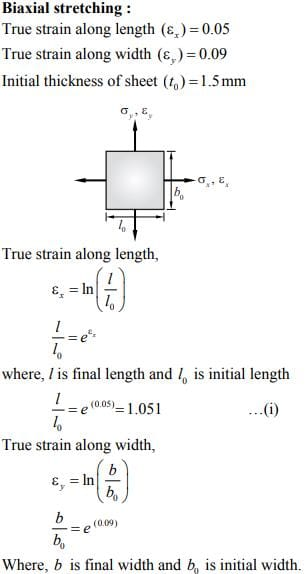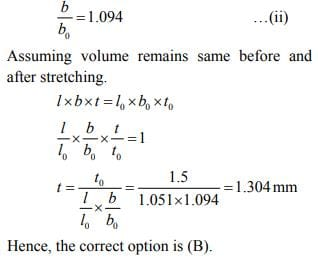Test: Stress & Strain - 1 - Question 7

A steel bar of 40 mm × 40 mm square cross-section is subjected to an axial compressive load of 200 kN. If the length of the bar is 2 m and E = 200 GPa, the elongation of the bar will be:

Detailed Solution for Test: Stress & Strain - 1 - Question 7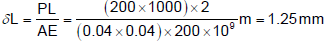Test: Stress & Strain - 1 - Question 8

The ultimate tensile strength of a material is 400 MPa and the elongation up to maximum load is 35%. If the material obeys power law of hardening, then the true stress-true strain relation (stress in MPa) in the plastic deformation range is:

Detailed Solution for Test: Stress & Strain - 1 - Question 8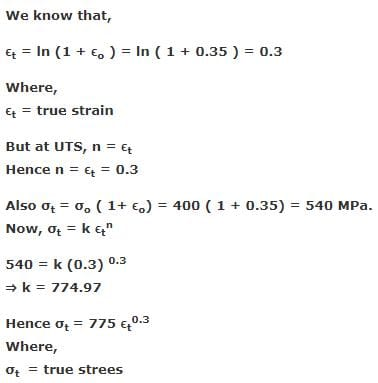Test: Stress & Strain - 1 - Question 9

An axial residual compressive stress due to a manufacturing process is present on the outer surface of a rotating shaft subjected to bending. Under a given bending load, the fatigue life of the shaft in the presence of the residual compressive stress is:

Detailed Solution for Test: Stress & Strain - 1 - Question 9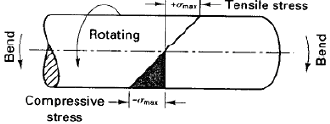A cantilever-loaded rotating beam, showing the normal distribution of surface stresses. (i.e., tension at the top and compression at the bottom)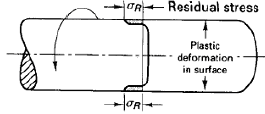The residual compressive stresses induced.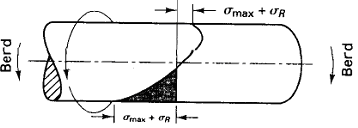Net stress pattern obtained when loading a surface treated beam. The reduced magnitude of the tensile stresses contributes to increased fatigue life.

Test: Stress & Strain - 1 - Question 10

A test specimen is stressed slightly beyond the yield point and then unloaded. Its yield strength will

Detailed Solution for Test: Stress & Strain - 1 - Question 10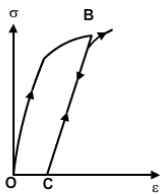Test: Stress & Strain - 1 - Question 11

The deformation of a bar under its own weight as compared to that when subjected to a direct axial load equal to its own weight will be:

Detailed Solution for Test: Stress & Strain - 1 - Question 11

Elongation of bar due to its own weight=Pl/2AE

Elongation of bar due to axial load=Pl/AE

Now comparing both..we will take the ratio.. (Pl/2AE)÷(Pl/AE)=1/2...i.e half

Test: Stress & Strain - 1 - Question 12

The phenomenon of slow growth of strain under a steady stress is called:

Detailed Solution for Test: Stress & Strain - 1 - Question 12

Consider a typical stress-strain curve of the material: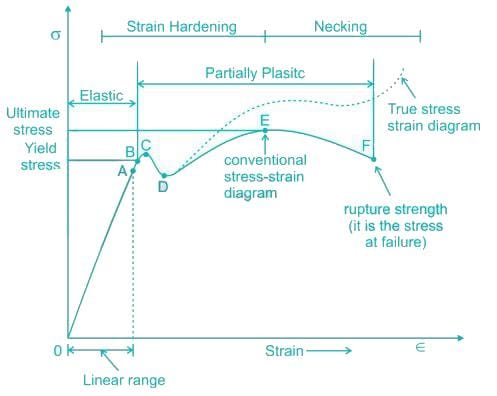1. Proportionality Limit: It is the limit where stress is directly proportional to strain i.e. Hook’s law is valid. (Point A in the diagram)
2. Elastic limit: It is the limit up to which material can regain its original position after unloading. (Point B in the diagram).
3. Yielding: The slow growth of strain or increase in strain under steady stress or load is called yielding. (region BC in the diagram)
4. Strain Hardening: After yielding, there is an increase in the deformation of material that starts again on an increase in load called strain hardening (region CD in the diagram).
5. Necking: It is deformation in material that continues even after slow removal of load. (Region DE in the diagram).
6. Fracture/Breaking: It is the point up to which material can sustain the load. If the load is applied beyond this point material will fail ultimately. (Point E in the diagram)
7. Creep is the tendency of a solid material to deform permanently under the influence of sustained load for a very large period of time.
Test: Stress & Strain - 1 - Question 13

A bolt of uniform strength can be developed by

Detailed Solution for Test: Stress & Strain - 1 - Question 13

A bolt of uniform strength can be developed by keeping the core diameter of threads equal to the diameter of the unthreaded portion of the bolt as Uniform strength means equal to threaded and unthreaded portion.

Test: Stress & Strain - 1 - Question 14

A bar of length L tapers uniformly from diameter 1.1 D at one end to 0.9 D at the other end. The elongation due to axial pull is computed using mean diameter D. What is the approximate error in computed elongation?

Detailed Solution for Test: Stress & Strain - 1 - Question 14

Actual elongation of the bar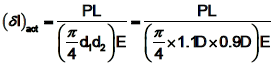Calculated elongation of the bar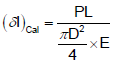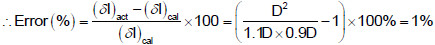Test: Stress & Strain - 1 - Question 15

In the case of an engineering material under unidirectional stress in the x -direction, the Poisson's ratio is equal to (symbols have the usual meanings)

Detailed Solution for Test: Stress & Strain - 1 - Question 15

Since stress is in x direction, longitudinal strain will develop in x direction. and lateral strain will be in y direction. Poisson's ratio= lateral strain/ longitudinal strain. i.e.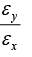Test: Stress & Strain - 1 - Question 16

If a piece of material neither expands nor contracts in volume when subjected to stress, then the Poisson‟s ratio must be

Detailed Solution for Test: Stress & Strain - 1 - Question 16

€v = 0. (1-2¥) × (£x + £y + £z) / E = 0.

Since body is constrained in all directions there will be equal stress in all directions.

£x = £y = £z

1-2¥ = 0

¥ = 1/2 = 0.5

Test: Stress & Strain - 1 - Question 17

Assertion (A): Plastic deformation is a function of applied stress, temperature and strain rate.

Reason (R): Plastic deformation is accompanied by change in both the internal and external state of the material.

Detailed Solution for Test: Stress & Strain - 1 - Question 17
• Following the elastic deformation, material undergoes plastic deformation.
• Also characterized by relation between stress and strain at constant strain rate and temperature.
• Microscopically…it involves breaking atomic bonds, moving atoms, then restoration of bonds.
• Stress-Strain relation here is complex because of atomic plane movement, dislocation movement, and the obstacles they encounter.
• Crystalline solids deform by processes – slip and twinning in particular directions.
• Amorphous solids deform by viscous flow mechanism without any directionality.
• Equations relating stress and strain are called constitutive equations.
• A true stress-strain curve is called flow curve as it gives the stress required to cause the material to flow plastically to certain strain.
Test: Stress & Strain - 1 - Question 18

The correct sequence of creep deformation in a creep curve in order of their elongation is :

Detailed Solution for Test: Stress & Strain - 1 - Question 18
• Primary or transient creep occurs first.
• For secondary creep, sometimes termed steady-state creep, the rate is constant; that is, the plot becomes linear, finally tertiary creep occurs in which there is an acceleration of the rate and ultimate failure.
Test: Stress & Strain - 1 - Question 19

Consider the following statements:

For increasing the fatigue strength of welded joints it is necessary to employ

1. Grinding

2. Coating

3. Hammer peening

Of the above statements

Detailed Solution for Test: Stress & Strain - 1 - Question 19

A polished surface by grinding can take more number of cycles than a part with rough surface.

In Hammer peening residual compressive stress lowers the peak tensile stress

Test: Stress & Strain - 1 - Question 20

The maximum energy that can be absorbed up to the elastic limit, without creating a permanent distortion is called

Detailed Solution for Test: Stress & Strain - 1 - Question 20

Proof resilience:

The strain energy stored in a body due to external loading, within the elastic limit, is known as resilience and the maximum energy which can be stored in a body up to the elastic limit is called proof resilience.

Modulus of resilience:

Modulus of resilience is defined as proof resilience per unit volume. It is the area under the stress-strain curve up to the elastic limit.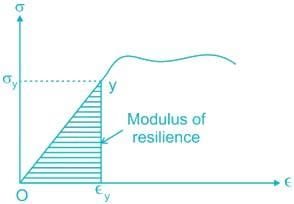Toughness:

It is defined as the ability of the material to absorb energy before fracture takes place.

• This property is essential for machine components which are required to withstand impact loads.
• Tough materials have the ability to bend, twist or stretch before failure takes place.
• Toughness is measured by a quantity called modulus of toughness. Modulus of toughness is the total area under the stress-strain curve in a tension test.

Flexure rigidity:

• It is a measure of the resistance of a beam to bending, that is, the larger the flexural rigidity, the smaller the curvature for a given bending moment.
• EI = Flexural rigidity

## GATE Mechanical (ME) 2024 Mock Test Series

27 docs|243 tests
Information about Test: Stress & Strain - 1 Page
In this test you can find the Exam questions for Test: Stress & Strain - 1 solved & explained in the simplest way possible. Besides giving Questions and answers for Test: Stress & Strain - 1, EduRev gives you an ample number of Online tests for practice

## GATE Mechanical (ME) 2024 Mock Test Series

27 docs|243 tests